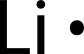# 8.1: Electron Dot Diagrams

$$\newcommand{\vecs}{\overset { \rightharpoonup} {\mathbf{#1}} }$$ $$\newcommand{\vecd}{\overset{-\!-\!\rightharpoonup}{\vphantom{a}\smash {#1}}}$$$$\newcommand{\id}{\mathrm{id}}$$ $$\newcommand{\Span}{\mathrm{span}}$$ $$\newcommand{\kernel}{\mathrm{null}\,}$$ $$\newcommand{\range}{\mathrm{range}\,}$$ $$\newcommand{\RealPart}{\mathrm{Re}}$$ $$\newcommand{\ImaginaryPart}{\mathrm{Im}}$$ $$\newcommand{\Argument}{\mathrm{Arg}}$$ $$\newcommand{\norm}{\| #1 \|}$$ $$\newcommand{\inner}{\langle #1, #2 \rangle}$$ $$\newcommand{\Span}{\mathrm{span}}$$ $$\newcommand{\id}{\mathrm{id}}$$ $$\newcommand{\Span}{\mathrm{span}}$$ $$\newcommand{\kernel}{\mathrm{null}\,}$$ $$\newcommand{\range}{\mathrm{range}\,}$$ $$\newcommand{\RealPart}{\mathrm{Re}}$$ $$\newcommand{\ImaginaryPart}{\mathrm{Im}}$$ $$\newcommand{\Argument}{\mathrm{Arg}}$$ $$\newcommand{\norm}{\| #1 \|}$$ $$\newcommand{\inner}{\langle #1, #2 \rangle}$$ $$\newcommand{\Span}{\mathrm{span}}$$$$\newcommand{\AA}{\unicode[.8,0]{x212B}}$$Figure $$\PageIndex{1}$$ (Credit: User:Veatchw/Wikipedia; Source: Commons Wikimedia, Veer vs 34(opens in new window) [commons.wikimedia.org]; License: Public Domain)

### How do we show electrons in atoms?

Diagrams contain a lot of useful information in a compact format. What does the diagram above tell us? The football play diagrammed above describes the lineup of each player on the team and describes how they will move when the ball is snapped. Diagrams of electrons give similar information about where certain electrons are. We can mark these electrons and indicate what happens to them when an element reacts.

## Electron Dot Diagrams

Recall that the valence electrons of an atom are the electrons located in the highest occupied principal energy level. Valence electrons are primarily responsible for the chemical properties of elements. The number of valence electrons can be easily determined from the electron configuration. Several examples from the second period elements are shown in the table below.

Table $$\PageIndex{1}$$:

Element Electron Configuration Number of Valence Electrons
lithium $$1s^2 \: 2s^1$$ 1
beryllium $$1s^2 \: 2s^2$$ 2
nitrogen $$1s^2 \: 2s^2 \: 2p^3$$ 5
neon $$1s^2 \: 2s^2 \: 2p^6$$ 8

In each case, valence electrons are those in the second principal energy level. As one proceeds left to right across a period, the number of valence electrons increases by one. In the $$s$$ block, Group 1 elements have one valence electron, while Group 2 elements have two valence electrons. In the $$p$$ block, the number of valence electrons is equal to the group number minus ten. Group 13 has three valence electrons, Group 14 has four, and so on...up through Group 18 with eight. The eight valence electrons, a full outer $$s$$ and $$p$$ sublevel, give the noble gases their special stability.

When examining chemical bonding, it is necessary to keep track of the valence electrons of each atom. Electron dot diagrams are diagrams in which the valence electrons of an atom are shown as dots distributed around the element's symbol. A beryllium atom, with two valence electrons, has the electron dot diagram below:Figure $$\PageIndex{2}$$ (Credit: Joy Sheng; Source: CK-12 Foundation; License: CC BY-NC-SA 3.0(opens in new window))

Since electrons repel each other, the dots for a given atom are distributed evenly around the symbol before they are paired. The table below shows the electron dot diagrams for the entire second period.

Table $$\PageIndex{2}$$: Electron Dot Diagrams for the Second Period Elements

Electron Dot Diagrams for the Second Period Elements
Group Number Electron Dot Diagram
1Figure $$\PageIndex{3}$$ (Credit: Joy Sheng; Source: CK-12 Foundation; License: CC BY-NC-SA 3.0(opens in new window))
2Figure $$\PageIndex{4}$$ (Credit: Joy Sheng; Source: CK-12 Foundation; License: CC BY-NC-SA 3.0(opens in new window))
13Figure $$\PageIndex{5}$$ (Credit: Joy Sheng; Source: CK-12 Foundation; License: CC BY-NC-SA 3.0(opens in new window))
14Figure $$\PageIndex{6}$$ (Credit: Joy Sheng; Source: CK-12 Foundation; License: CC BY-NC-SA 3.0(opens in new window))
15Figure $$\PageIndex{7}$$ (Credit: Joy Sheng; Source: CK-12 Foundation; License: CC BY-NC-SA 3.0(opens in new window))
16Figure $$\PageIndex{8}$$ (Credit: Joy Sheng; Source: CK-12 Foundation; License: CC BY-NC-SA 3.0(opens in new window))
17Figure $$\PageIndex{9}$$ (Credit: Joy Sheng; Source: CK-12 Foundation; License: CC BY-NC-SA 3.0(opens in new window))
18Figure $$\PageIndex{10}$$ (Credit: Joy Sheng; Source: CK-12 Foundation; License: CC BY-NC-SA 3.0(opens in new window))

Electron dot diagrams would be the same for each element in the representative element groups. Most transition elements have two valence electrons, though some that have unusual electron configurations have only one.

## Summary

• Electron dot diagrams show the valence electrons for an atom.
• The dot diagrams are the same for each element in the representative element groups.

## Review

1. What are valence electrons primarily responsible for?
2. Calcium would have the same electron dot structure as which element pictured in the table?
3. What is the symbol for an element that would have the same electron dot structure as carbon?
4. Would you expect the group 18 elements to have the same electron dot diagram as neon?

This page titled 8.1: Electron Dot Diagrams is shared under a CK-12 license and was authored, remixed, and/or curated by CK-12 Foundation via source content that was edited to the style and standards of the LibreTexts platform; a detailed edit history is available upon request.